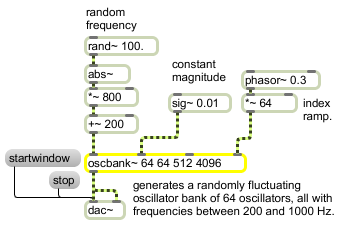oscbank~

Non-interpolating oscillator bank

Description

oscbank~ is a non-interpolating oscillator bank with signal inputs to set oscillator frequency and magnitude.

Arguments

Name Type Opt Description
number-of-oscillators int opt The number of oscillators.
frequency-smoothing-factor (samples) int opt The number of samples across which frequency smoothing is done.
amplitude-smoothing-factor (samples) int opt The number of samples across which amplitude smoothing is done.
lookup-table-size (samples) int opt The size, in samples, of the sinewave lookup table used by the oscbank~ object. The default is 4096. Since oscbank~ uses uninterpolated oscillators, you can choose to use a sinetable of larger size at the expense of CPU.

Note: There is only one wavetable for all oscillators in a given oscbank~ object,

Messages

 int inlet-specific-parameter-settings [int] Performs the same function as float in any inlet (see signal and float messages). float oscillator-phase (0.0 through 1.0) [float] Performs the same function as a signal according to each inlet. clear The word clear sets the frequency of all oscillators to zero and zeros all amplitudes. copybuf buffer-object-name [symbol]offset (samples) [int] In left inlet: The word copybuf, followed by a symbol that specifies a buffer, copies samples from the buffer into the oscbank~ object's internal wavetable. The number of samples is set using the tabpoints message. An optional second integer argument specifies the position in the buffer at which samples are loaded (offset). framesync behavioral-flag (0 or nonzero) [int] The word framesync, followed by a non-zero number, enables frame synchronous operation. When frame synchronous operation is enabled, a given index's values will only change or begin their interpolated ramps to the next value when the index input signal is 0 (or once per n sample frame). Otherwise, a given index's values will change or begin their interpolated ramps to the next value when the index input signal is equal to that index. The default is off. freqsmooth frequency-smoothing-factor (samples) [int] The word freqsmooth, followed by an int, sets the number of samples across which frequency smoothing is done. The default is 1 (no smoothing). magsmooth amplitude-smoothing-factor (samples) [int] The word magsmooth, followed by an int, sets the number of samples across which magnitude (amplitude) smoothing is done on a oscillator. The default is 0 (no amplitude smoothing). set frequency-amplitude-pairs (floats) [list] The word set, followed by pairs of floating-point values, sets the frequency and amplitude of an oscillator in the oscillator bank. A list of n pairs will set the first n oscillators in the oscbank~ object and zero the amplitude of all others. silence The word silence zeros the amplitude of all the oscillators. size number-of-oscillators [int] The word size, followed by a number, sets the number of oscillators. The default is 64. tabpoints lookup-table-size (samples) [int] The word tabpoints, followed by a number, sets the number of wavetable points (samples) in the oscbank~ object's internal wavetable. The default is 4096. The number of wavetable points should be a power or two between 2 2 and 2 16 . Any other value will be rounded to the nearest power of two. signal In left inlet: Sets the frequency of the oscillator whose index is currently referenced to the current floating-point value of the signal. The default value is 0. In 2nd inlet: Sets the magnitude (amplitude) of the oscillator whose index is currently referenced. In 3rd inlet: If framesync is enabled using the framesync 1 message, a signal in the range 0-1.0 sets the phase of the oscillator currently being referenced. In 4th inlet: Sets the index of the oscillator currently being referenced.

Output

signal: A waveform consisting of the sum of the specified frequencies and amplitudes.

Examplesoscbank~ creates a bank of oscillators that you can control with one object